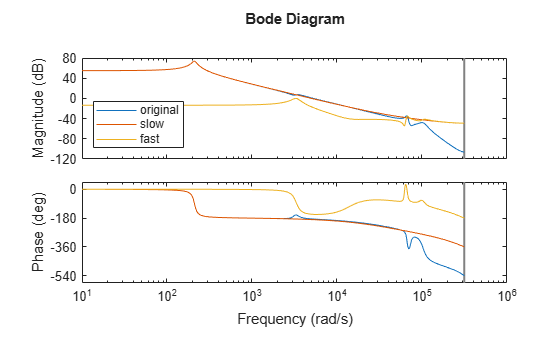# freqsep

Slow-fast decomposition

## Syntax

``````[Gs,Gf] = freqsep(G,fcut)``````
``````[Gs,Gf] = freqsep(G,fcut,options)``````

## Description

example

``````[Gs,Gf] = freqsep(G,fcut)``` decomposes a linear dynamic system into slow and fast components around the specified cutoff frequency. The decomposition is such that `G` = `Gs` + `Gf`.```

example

``````[Gs,Gf] = freqsep(G,fcut,options)``` specifies additional options for the decomposition. ```

## Examples

collapse all

```load numdemo Pd bode(Pd)````Pd` has four complex poles and one real pole. The Bode plot shows a resonance around 210 rad/s and a higher-frequency resonance below 10,000 rad/s.

Decompose this model around 1000 rad/s to separate these two resonances.

```[Gs,Gf] = freqsep(Pd,10^3); bode(Pd,Gs,Gf) legend('original','slow','fast','Location','Southwest')```The Bode plot shows that the slow component, `Gs`, contains only the lower-frequency resonance. This component also matches the DC gain of the original model. The fast component, `Gf`, contains the higher-frequency resonances and matches the response of the original model at high frequencies. The sum of the two components `Gs+Gf` yields the original model.

Decompose a model into slow and fast components between poles that are closely spaced.

The following system includes a real pole and a complex pair of poles that are all close to s = -2.

`G = zpk(-.5,[-1.9999 -2+1e-4i -2-1e-4i],10);`

Try to decompose the model about 2 rad/s, so that the slow component contains the real pole and the fast component contains the complex pair.

`[Gs,Gf] = freqsep(G,2);`
```Warning: One or more fast modes could not be separated from the slow modes. To force separation, increase the absolute or relative tolerances ("AbsTol" and "RelTol" options). Type "help freqsepOptions" for more information. ```

These poles are too close together for `freqsep` to separate. Increase the relative tolerance to allow the separation.

```options = freqsepOptions('RelTol',1e-4); [Gs,Gf] = freqsep(G,2,options);```

Now `freqsep` successfully separates the dynamics about 2 rad/s.

`slowpole = pole(Gs)`
```slowpole = -1.9999 ```
`fastpole = pole(Gf)`
```fastpole = 2×1 complex -2.0000 + 0.0001i -2.0000 - 0.0001i ```

## Input Arguments

collapse all

Dynamic system to decompose, specified as a numeric LTI model, such as a `ss` or `tf` model.

Cutoff frequency for fast-slow decomposition, specified as a positive scalar. The output `Gs` contains all poles with natural frequency less than `fcut`. The output `Gf` contains all poles with natural frequency greater than or equal to `fcut`.

Options for the decomposition, specified as an options set you create with `freqsepOptions`. Available options include absolute and relative tolerance for accuracy of the decomposed systems.

## Output Arguments

collapse all

Slow dynamics of the decomposed system, returned as a numeric LTI model of the same type as `G`. `Gs` contains all poles of `G` with natural frequency less than `fcut`, and is such that `G` = `Gs` + `Gf`.

Fast dynamics of the decomposed system, returned as a numeric LTI model of the same type as `G`. `Gf` contains all poles of `G` with natural frequency greater than or equal to `fcut`, and is such that `G` = `Gs` + `Gf`.

Model Reducer